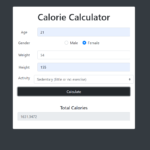## Calorie Calculator In JavaScript With Source code

The Calorie Calculator is a simple project developed using JavaScript, CSS, and HTML. This project is an interesting project. The user can calculate the calorie amount that they can intake in a day for a healthy living.

Type : Project

File Size : 22.8 KB# Similar Projects and Reports

#### Calorie Calculator In JavaScript With Source code

The Calorie Calculator is a simple project developed using JavaScript, CSS, and HTML. This project is an interesting project. The user can calculate the calorie amount that they can intake in a day for a healthy living.

#### Calculator In JavaScript With Source Code

The calculator is a simple project in HTML5, CSS, and JavaScript. Here, the calculator functions as that of a real-life simple calculator. This project is for solving the mathematical calculations of numbers. You can see the basic features of the calculat...

#### JavaScript Calculator With Source Code

This javascript calculator project is a simple project in HTML5, CSS, and JavaScript. Here, the calculator functions as that of a real-life simple calculator. This project is for solving the mathematical calculations of numbers. You can see the basic feat...

#### Tip Calculator In Javascript With Source Code

The Tip Calculator is a simple project developed using JavaScript, CSS, and HTML. This project is an interesting project. The user can calculate the tip amount that they can get from a single bill pay.

#### BMI CALCULATOR IN JAVASCRIPT WITH SOURCE CODE

BMI Calculator is a simple project developed using HTML5, CSS, and JavaScript. This project is for checking your BMI. With the BMI value, you can check whether you have a healthy weight or not. Also, you can see the basic features of this calculator in th...

#### LOVE CALCULATOR IN JAVASCRIPT WITH SOURCE CODE

Love Calculator is a simple project in HTML5, CSS, and JavaScript. This is interesting project made for fun. This project is for finding the compatibility calculations between two peoples. Also, you can see the basic features of the love calculator in thi...